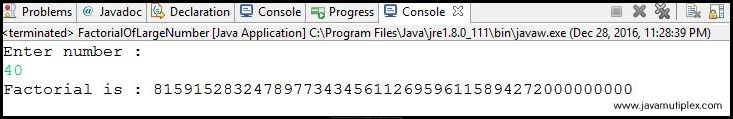## Problem Description :

Write a program in Java that calculates factorial of a large number.

## Concept :

The factorial of non-negative integer is denoted by n! and it is the product of all positive integers less than or equal to n.  For example - 5! = 5 X 4 X 3 X 2 X 1 = 120.
For calculating factorial, we are using BigInteger class that is present in java.math.* package.

## Output :Output - How to calculate factorial of a very large number in Java?

## References :

Thank you friends, I hope you have clearly understood the solution of this problem. If you have any doubt, suggestion or query please feel free to comment below. You can also discuss this solution in our forum.

Tags : Calculate factorial in Java, Solution in Java, large number, BigInteger, if else statement, while loop, String.How to calculate factorial of large number in Java?Reviewed by Rohit Agarwal on 12/28/2016 Rating: 5